# Division With Remainders (2-Digit Quotients)

Times up!
Times up! You may finish answering the current question and then proceed to your score!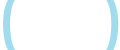Sorry, you have used all of your available hints for this lesson.
Practice Limit Reached

You've reached your daily practice limit of 12 questions.

Award InformationAwarded toQuestion HintChoose Family Member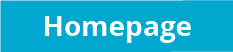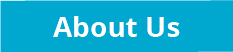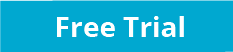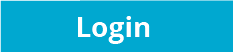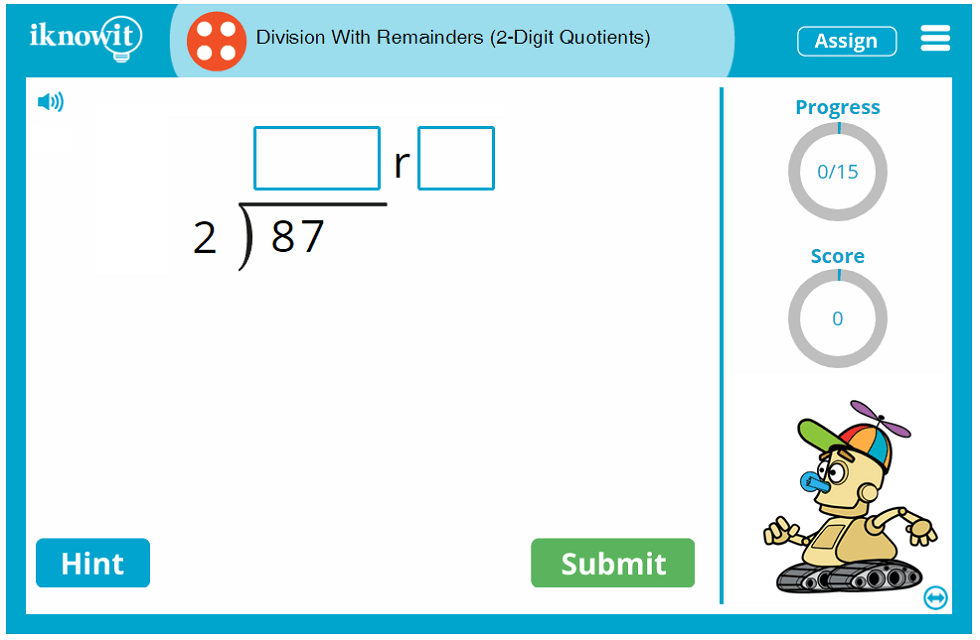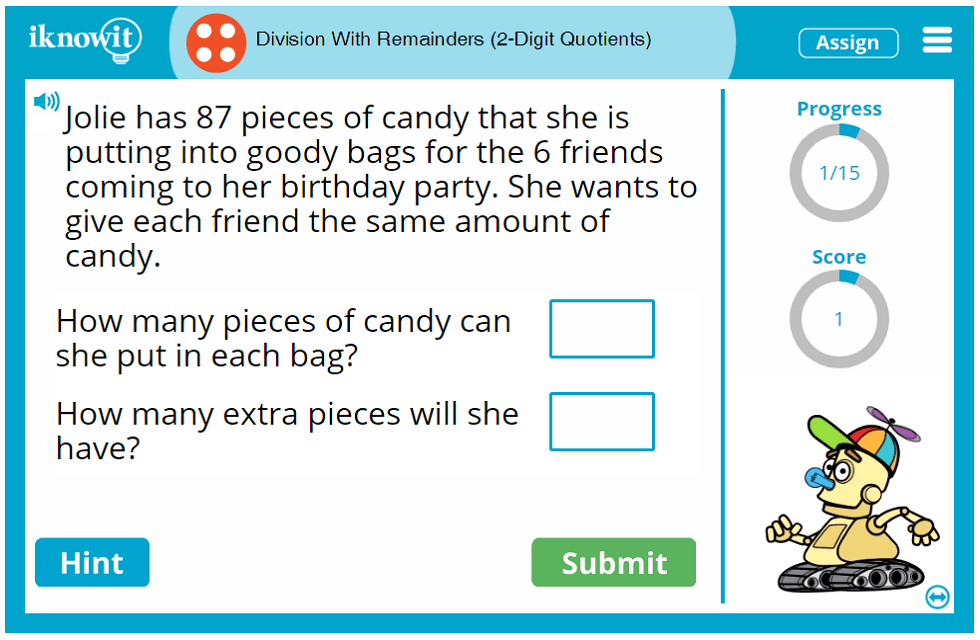## Interactive Math Game – Division with Remainders and 2-Digit Quotients

Children will practice basic division with remainders and two-digit quotients in this fourth grade-level math lesson from "I Know It". Math problems are given in a variety of question formats, including word problems. After completing this online math lesson, your students will have a greater understanding of basic division skills.

If you're wondering what kind of problems your students can expect in this interactive math lesson on division, here are some examples: "Jordan has 62 t-shirts that he has folded. He wants to put an equal number of t-shirts in his dresser drawers. How many t-shirts can fit in each drawer? How many t-shirts will be left over?" and "Find the quotient and the remainder of 93 divided by 5."

When your students need a little extra help solving a math problem, they can click on the "Hint" button. They will be prompted to do the first step, divide the tens. When students answer a question incorrectly, they will be shown a detailed explanation page demonstrating how to solve the problem correctly: "Divide the tens. Bring down the ones. Divide the ones. Write the remainder."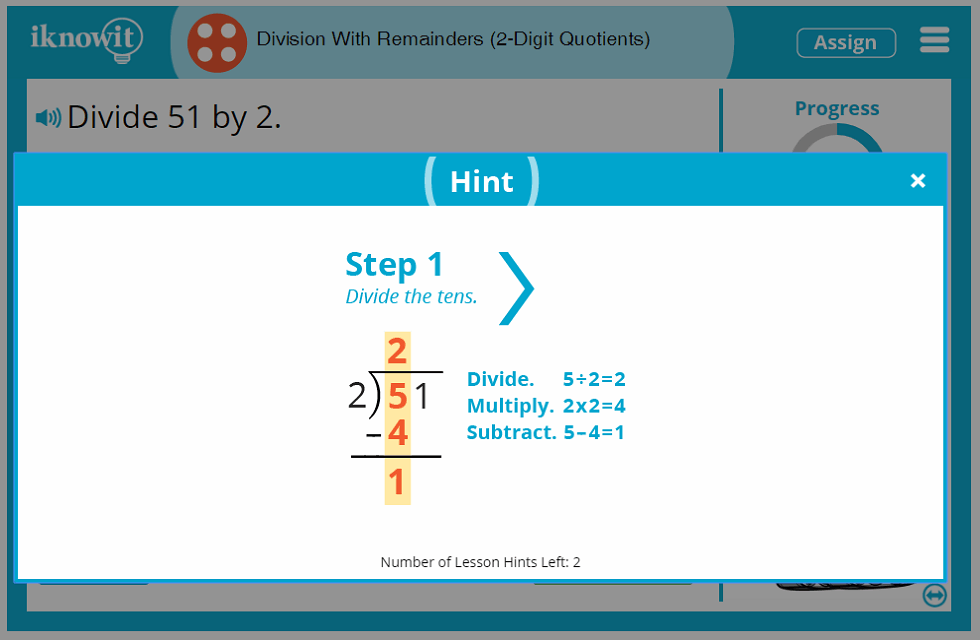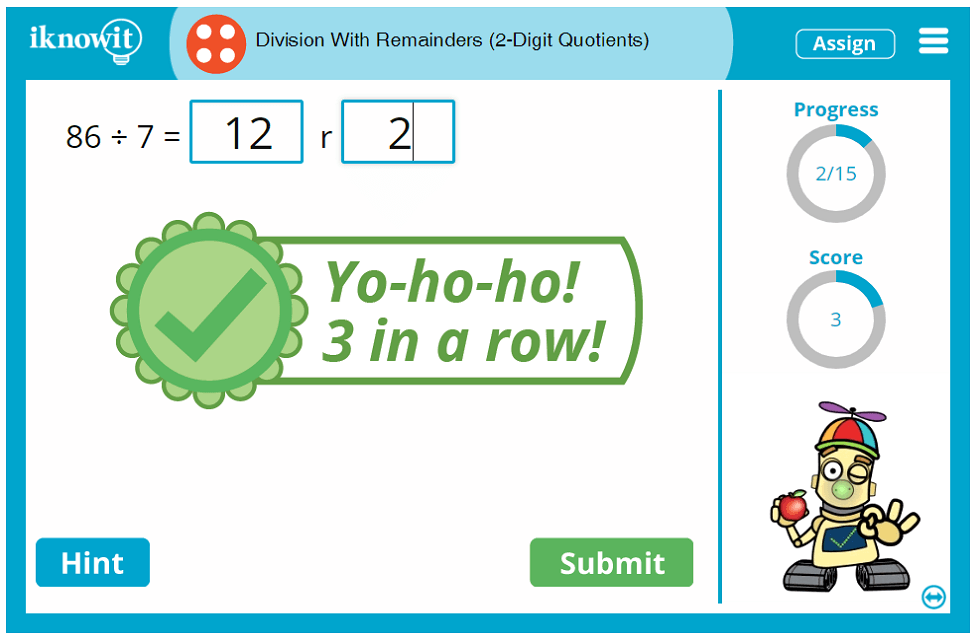## More About 'I Know It' Math Lessons

We built the "I Know It" math program because we are passionate about helping children improve their math skills in a way that is engaging, challenging, and fun. Students and teachers alike enjoy using our program. Children like the kid-friendly website layout, bold colors, big icons, and whimsical animated characters that cheer them on when they answer a math question correctly. Teachers appreciate the quality and scope of our lessons, plus the wide variety of elementary math topics our website covers.

Some additional features that educators and students like in our "I Know It" math lessons include the progress-tracker, which shows students how many questions they have answered in the lesson so far, the score tracker, which tells students how many questions they've answered correctly, and the speaker icon, which allows the question to be read aloud in a clear voice.

It is our hope that you and your class will try out this dividing with remainders lesson from "I Know It" today! Be sure to explore our other math topics and discover why there is so much to love about the "I Know It" math program.

## Free Trial and Membership Options

Please consider signing up for a free sixty-day trial of "I Know It" so you can try out this fourth grade division game with your class today! Your students will be able to try this lesson, as well as any lesson on our website, at no cost. Please know that students are capped at a total of twenty-five questions per day across all math lessons on "I Know It" in the free trial mode. If you would like unlimited access to our math lessons, you will need to become a paying member of the website.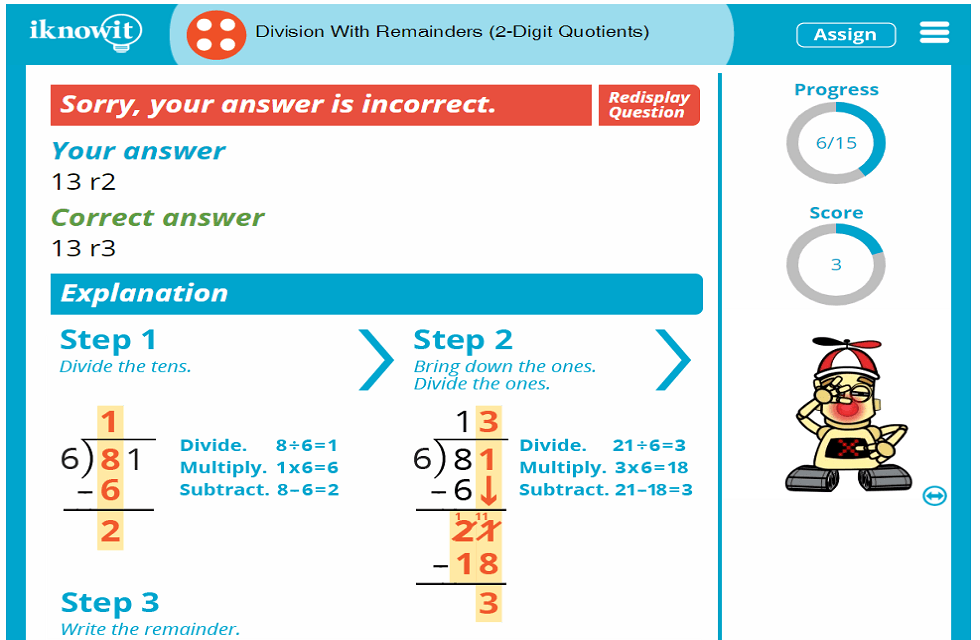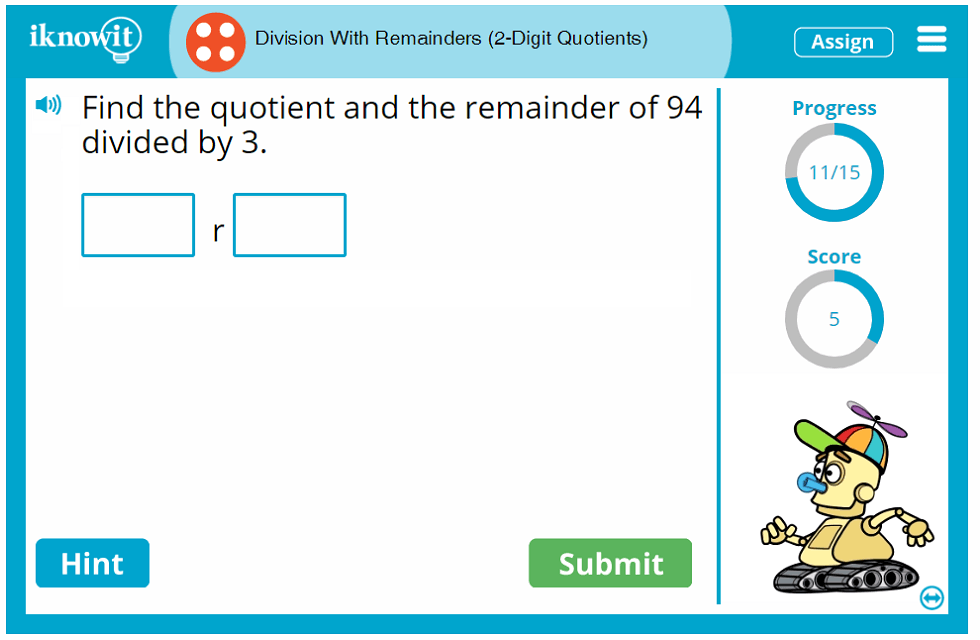## Level

This Level D division lesson may be ideal for your fourth grade class.

## Common Core Standard

4.NBT.6
Number and Operations in Base Ten

Students will use their understanding of place value and properties of operations to find whole number quotients and remainders with up to four-digit dividends and one-digit divisors.

## You might also be interested in...

Division: Three-Digit Quotients (Level D)
In this fourth grade-level math lesson, students will practice dividing numbers with three-digit quotients. Students will divide the hundreds, divide the tens, and divide the ones to obtain the three-digit quotient.

Dividing Money (Level D)
Students will practice dividing money in this fourth grade-level math activity. Questions are presented in a variety of formats, including word problems.